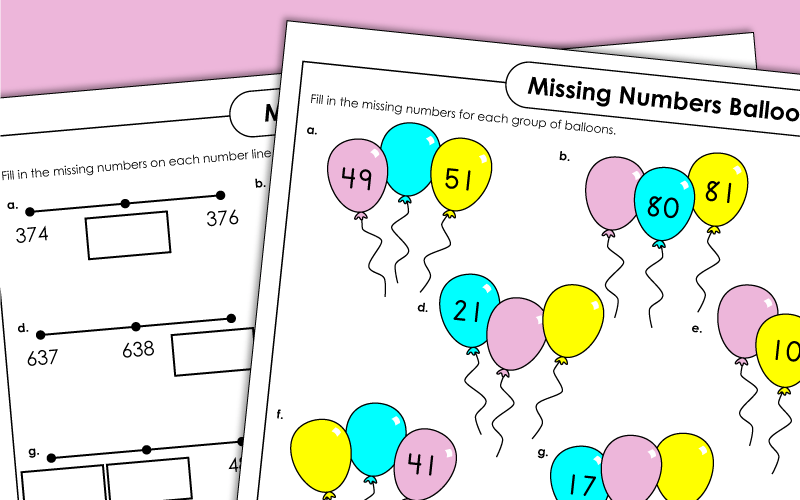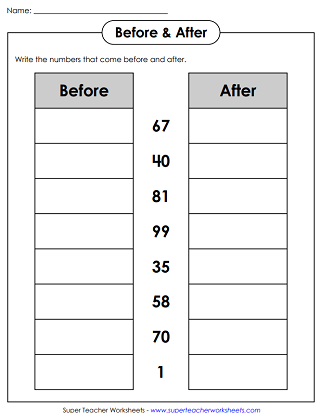# Counting Worksheets

Printable worksheets for counting 2, 3, and 4-digit numbers.  Includes counting on, counting backwards, and number line counting.## Counting: 2-Digit Numbers

With this pig-themed worksheet, students will practice counting from 1 to 100.
Travel through space by continuing the number sequence starting at number 48 and ending at 67. Write the missing numbers in the circles.
Students must fill in the empty boxes to complete each number lines.
Fill in the number line by writing the missing 2-digit numbers.
Penguins with numbers on their bellies are lined up in groups of three. Students must write the missing numbers on the penguins.
Count by 10 to find how many sticks there are together in all of the bundles. Then count the individual sticks and add the numbers together to find how many sticks are shown altogether.
This worksheet can help kids practice counting up to 100.
Students must read the given number and tell what comes before and after.  They also have to add ten and subtract ten.
Some balloons have numbers and others don't.  Complete the number sequence by filling in the missing numbers.
Students must read the given number and tell what comes before and after.  They also have to add ten and subtract ten.
Present this award to students who can count all the way up to 100.

## Counting: 3-Digit Numbers

Students are shown a number line with some numbers missing.  They must fill in the missing numbers.
This worksheet pictures clusters of penguins floating on ice.  Some penguins have numbers on them.  Students must use their counting skills to write numbers on the penguins without numbers.
Students must read the given number and tell what comes before and after.  They also have to add ten and subtract ten.
Fill in the balloons with the missing numbers to complete the counting sequence.
Students must read the given number and tell what comes before and after.  They also have to add ten and subtract ten.

## Counting 4-Digit Numbers

Students are given a number. They must add 1, 10, and 100 to the number. They must also subtract 1, 10, and 100.
Complete the number lines by filling in the missing numbers on each set of balloons.

## Also on Super Teacher Worksheets...

Counting up 10, 20, or 30

Looking for more basic counting worksheets?  This page has worksheets for teaching students to count up to 20.

Hundreds Charts

A whole page of super teacher worksheets dedicated to hundreds charts, ninety-nine charts, and place value charts.

Color by Number Worksheets

Simple color-by-number mystery pictures for students of any age.

Place Value Worksheets

Worksheets for teaching two-digit through seven-digit place value skills.

## Sample Worksheet ImagesMy Account
Site Information